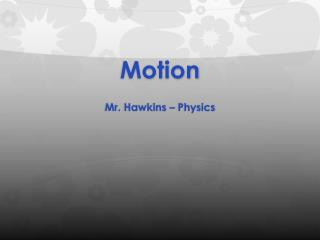DownloadDownload PresentationMotion

# Motion

Télécharger la présentation## Motion

- - - - - - - - - - - - - - - - - - - - - - - - - - - E N D - - - - - - - - - - - - - - - - - - - - - - - - - - -
##### Presentation Transcript

1. Motion Mr. Hawkins – Physics

2. F= Newton It is equal to the force that would give a mass of one kilogram an acceleration of one meter per second per second

3. Acceleration Units of m/sec2 meters/second/second Meters per second for every second

4. Force in Newton F=ma F=Newton (N) m=mass (Kg) a= acceleration m/sec2

5. Acceleration & Lab Use TWO photogates a= (0.05m/tb)-(0.05m/ta) tab

6. Example a= (0.05m/tb)-(0.05m/ta) tab a= (0.05m/.0296s)-(0.05m/.0447s) .2720s Acceleration= 2.1 m/sec2 or m/sec/sec

7. Force in Newton F=ma F=Newton (N) m=mass (Kg) a= acceleration m/sec2 0.7N .28Kg = 2.5 m/sec2 or m/sec/sec

8. Comparison of Both a= (0.05m/.0296s)-(0.05m/.0447s) .2720s Acceleration= 2.1 m/sec2 or m/sec/sec 0.7N .28Kg = 2.5 m/sec2 or m/sec/sec

9. There Different? Acceleration= 2.1 m/sec2 or m/sec/sec 2.1 m/sec2 – 2.5 m/sec2 2.5 m/sec2 0.7N .28Kg = 2.5 m/sec2 or m/sec/sec * 100 = -16% Error